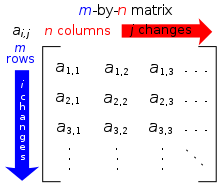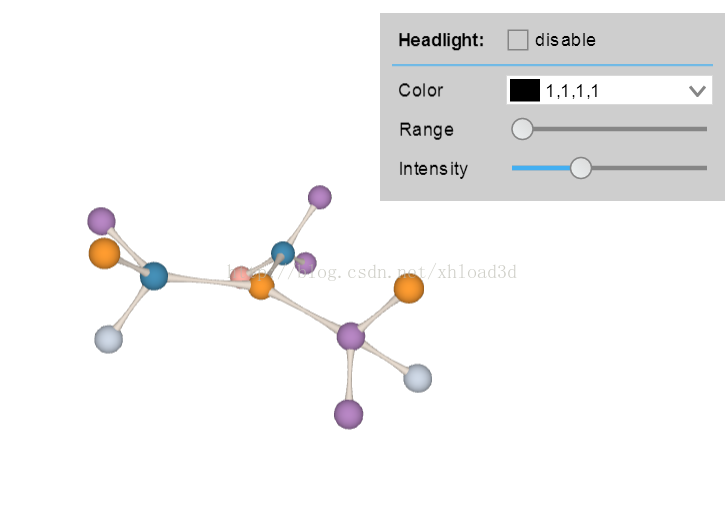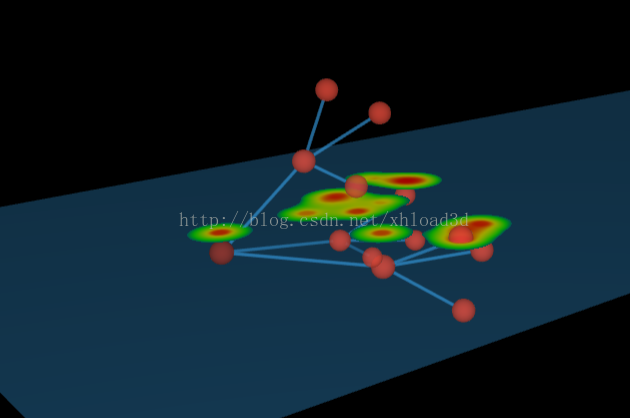# 通过矩阵变化实现的 3D 模型自动布局http://www.hightopo.com/demo/...``````<script src="../../guide/lib/core/ht.js"></script>
<script src="../../guide/lib/plugin/ht-forcelayout.js"></script>
<script src="../../guide/lib/plugin/ht-form.js"></script>``````

``````var colorList = ['#FFAFA4', '#B887C5', '#B9EA9C', '#CFD9E7', '#4590B8', '#FF9C30'],
colorLen = colorList.length;
var randomColor = function() {
var ran = Math.random() * colorLen;
return colorList[Math.floor(ran)]; // 随机6种颜色
};``````

``````var createNode = function(dm) { // 创建 node 节点 圆
var node = new ht.Node();
node.s({
'shape3d': 'sphere',
'shape3d.color': randomColor()
});
node.s3(40, 40, 40);
return node;
};``````

``````ht.Default.setShape3dModel( // 创建模型 根据 xy 平面的曲线，环绕一周形成 3D 模型。
'custom', ht.Default.createRingModel( [0.5, 0.5, -0.2, 0, 0.5, -0.5], [1, 3] )
);``````

HT 将用户自定义的属性和 HT 默认的属性调用方法分为 node.a 和 node.s 这样就能将两者有效地区分开来，我们在创建管线的时候就调用了这种方法：

``````var updatePipeline = function(edge) { // 重新设置 edge 的样式
var pipeline = edge.a('pipeline');
pipeline.s3(1, 1, 1);
pipeline.p3(0, 0, 0);

var node1 = edge.getSourceAgent(), // 获取图形上连接的起始节点
node2 = edge.getTargetAgent();
pipeline.s('mat', createMatrix(node1.p3(), node2.p3(), 20)); // 3d 整体图形矩阵变化
};``````

``````var createMatrix = function(p1, p2, width) { // createMatrix(array, matrix) 将一组 JSON 描述的缩放、移动和旋转等操作转换成对应的变化矩阵
var vec = [p2-p1, p2-p1, p2-p1],
dist = ht.Default.getDistance(p1, p2); // 获取两点之间距离，或向量长度
return ht.Default.createMatrix({
s3: [width, dist, width],
r3: [Math.PI/2 - Math.asin(vec/dist), Math.atan2(vec, vec), 0],
rotationMode: 'xyz',
t3: [(p1+p2)/2, (p1+p2)/2, (p1+p2)/2]
});
};``````

``````var createEdge = function(dm, node1, node2) { // 创建‘custom’模型的 edge
var node = new ht.Node();
node.s({
'shape3d': 'custom',
'shape3d.color': '#ECE0D4',
'layoutable': false
});

var edge = new ht.Edge(node1, node2);
edge.a('pipeline', node);
edge.s('edge.color', 'rgba(0, 0, 0, 0)');
return edge;
};````````````var formPane = new ht.widget.FormPane();
formPane.setWidth(230);
formPane.setHeight(125);

``````['Color', 'Range', 'Intensity'].forEach(function(name) {
var obj = { id: name },
func = function(oV, nV) {
};
if (name === 'Color')
obj.colorPicker = { // ht.widget.ColorPicker为颜色选择框
instant: true,
onValueChanged: func
};
else
obj.slider = { // 滑动条
min: 0,
max: name === 'Range' ? 20000 : 3,
step: 0.1,
onValueChanged: func
};
formPane.addRow([ name, obj ], [ 70, 0.1 ]);
});``````

slider 和 colorPicker 都是 HT 自定义的滑动条和颜色选择器，详情请参考 HT for Web 表单手册

172 声望
23 粉丝
0 条评论